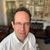Announcements
The way to achieve your own success is the willingness to help somebody else. Go for it!
cancel
Showing results for
Did you mean:Not applicable

## Total in staright table

Hi

I have the below straight table

The formula for the total column is  (days*volume*(0.3))+(days*volume)/150  = total

 employee id days volume total a 1 27 0.020116677 0.004707302 a 2 3 0.024473813 0.000636319 a 3 94 0.02513826 0.020479303

My output should be as below

 employee total a 0.025822924

i'm using the below formula i'm getting correct answer if i'm adding the ID field, but i dont want to show ID field and a unique employee

= sum(days* volume*0.3)+ sum(days)*sum( distinct volume) /150

1 Solution

Accepted SolutionsMVP

Try this:

=Sum(Aggr(Sum(days* volume*0.3) + Sum(days) * Sum(DISTINCT volume) /150, employee, id))

9 RepliesMVP

Try this:

=Sum(Aggr(Sum(days* volume*0.3) + Sum(days) * Sum(DISTINCT volume) /150, employee, id))Not applicable
Author

Hi Sunny its giving me very high value when compared to the actual value

its giving me 23.050 but the value is 0.65143....

employeeiddaysvolumetotal
a19830.764653784219

 0.651434MVP

Would you be able to share a sample where this isn't working?Creator

Hi John, are you pre-calculating the total amount in your load script?

If not, I would suggest that you consider moving this calculation to the script.

If you're wanting to calculate it in a chart, then I would imagine an expression like the one below would suffice:

sum(((days*volume*0.3)+(days*volume))/150)S.MVP

Try to create one variable for 0.3 like

LET Vstatic = num('0.3','##.##0')

Then use this variable in to that expression

Before develop something, think If placed (The Right information | To the right people | At the Right time | In the Right place | With the Right context)Creator III

Hi John,

Try this..

=Sum(Aggr((Sum(days* volume*0.3) + Sum(days) * Sum(DISTINCT volume)) /150,Employee,Id))Specialist

Sunny T's approach would work if the data was just those 3 rows. I suspect that this is not the raw data (the DISTINCT is the main clue).

If my suspicion is correct, please describe or show us the raw dataNot applicable
Author

Hi sunny tanks a lot and the problem is resolved nowNot applicable
Author